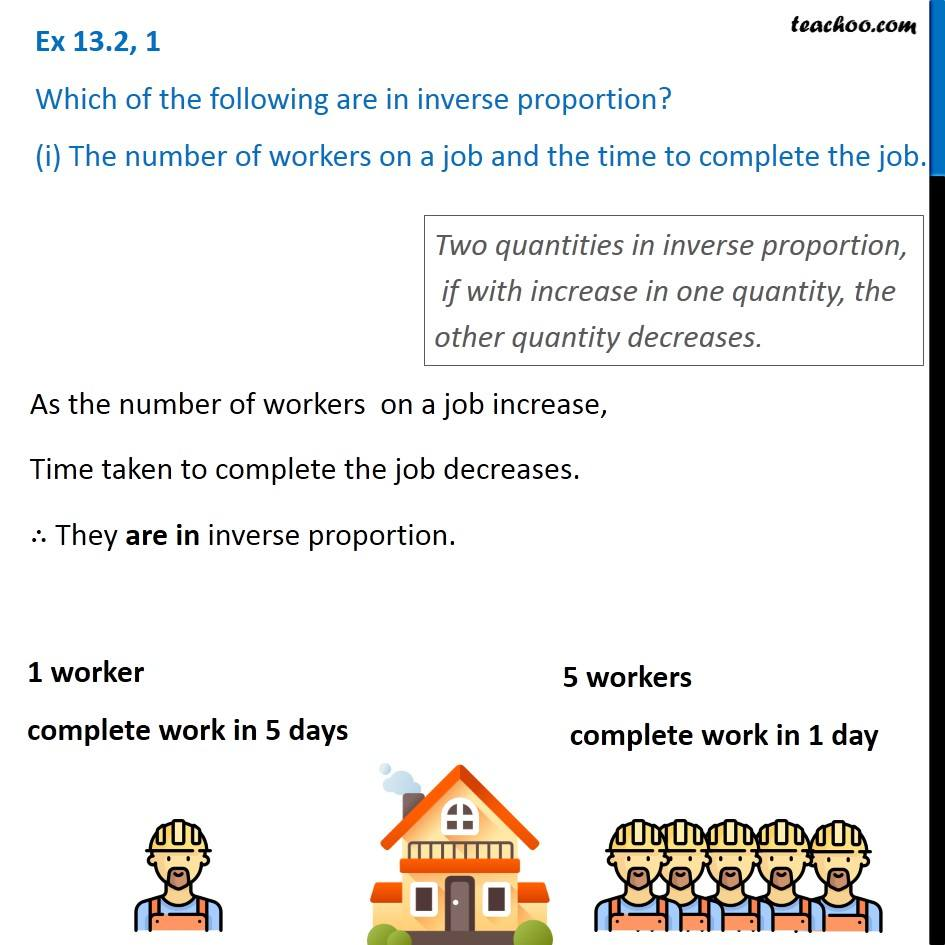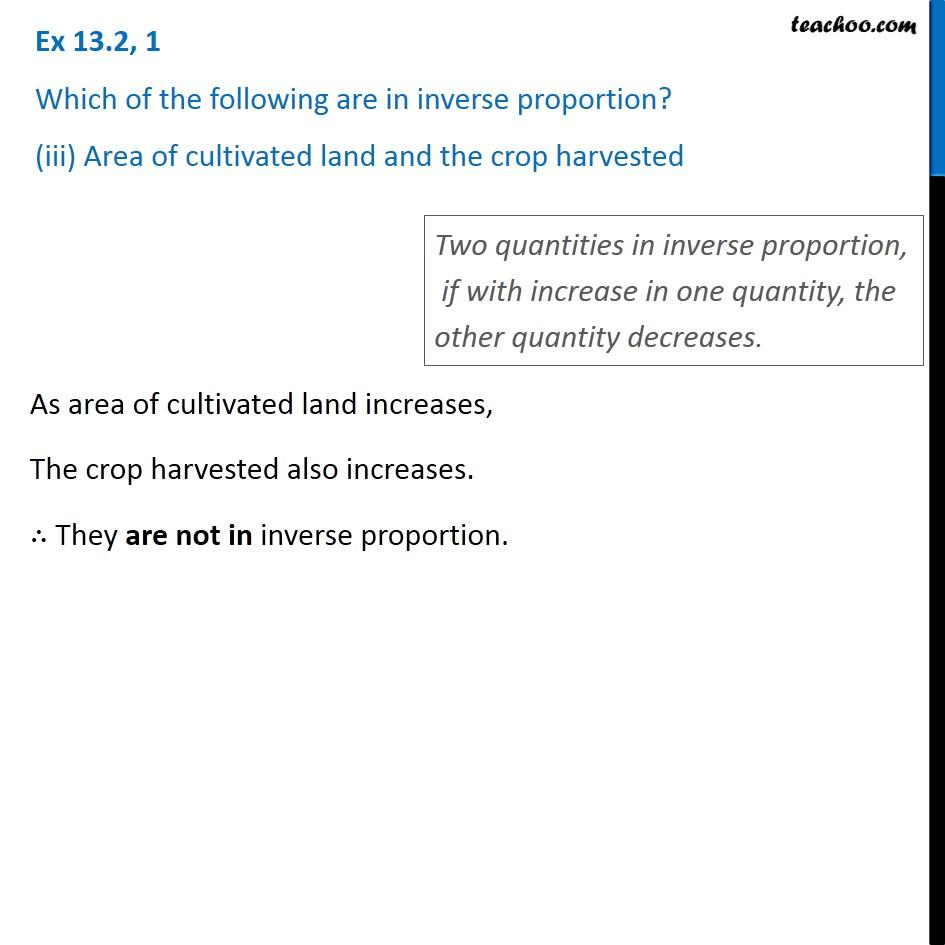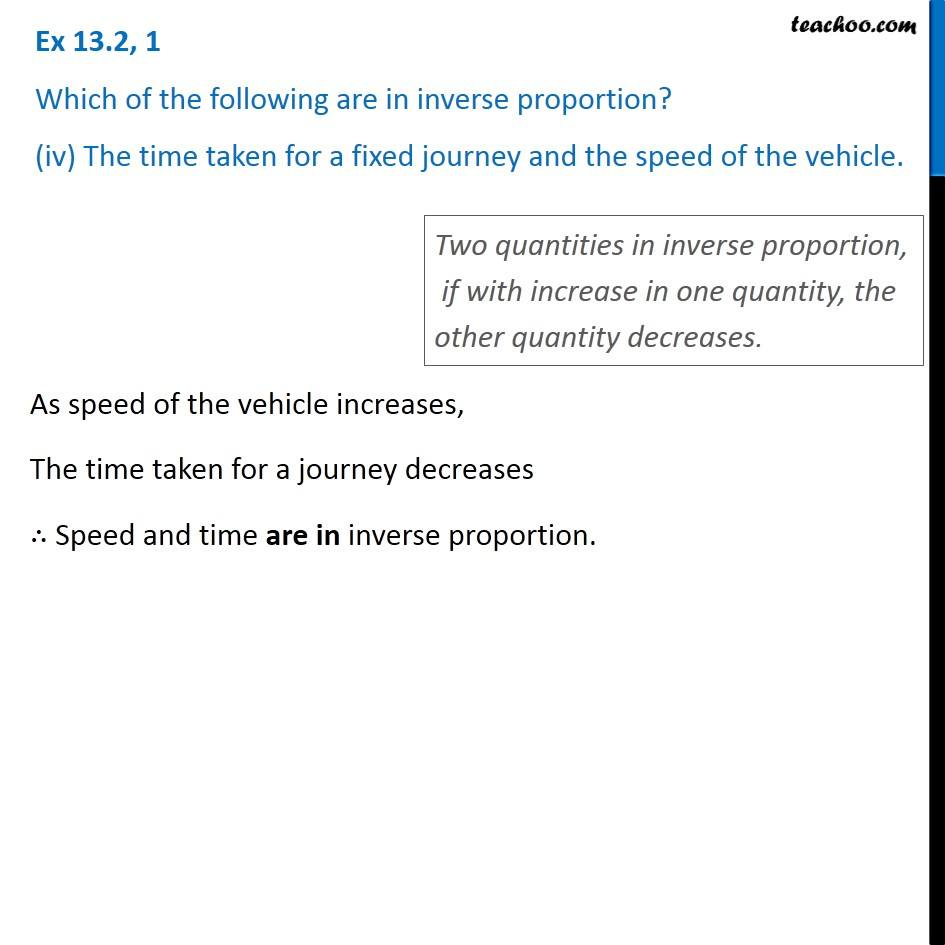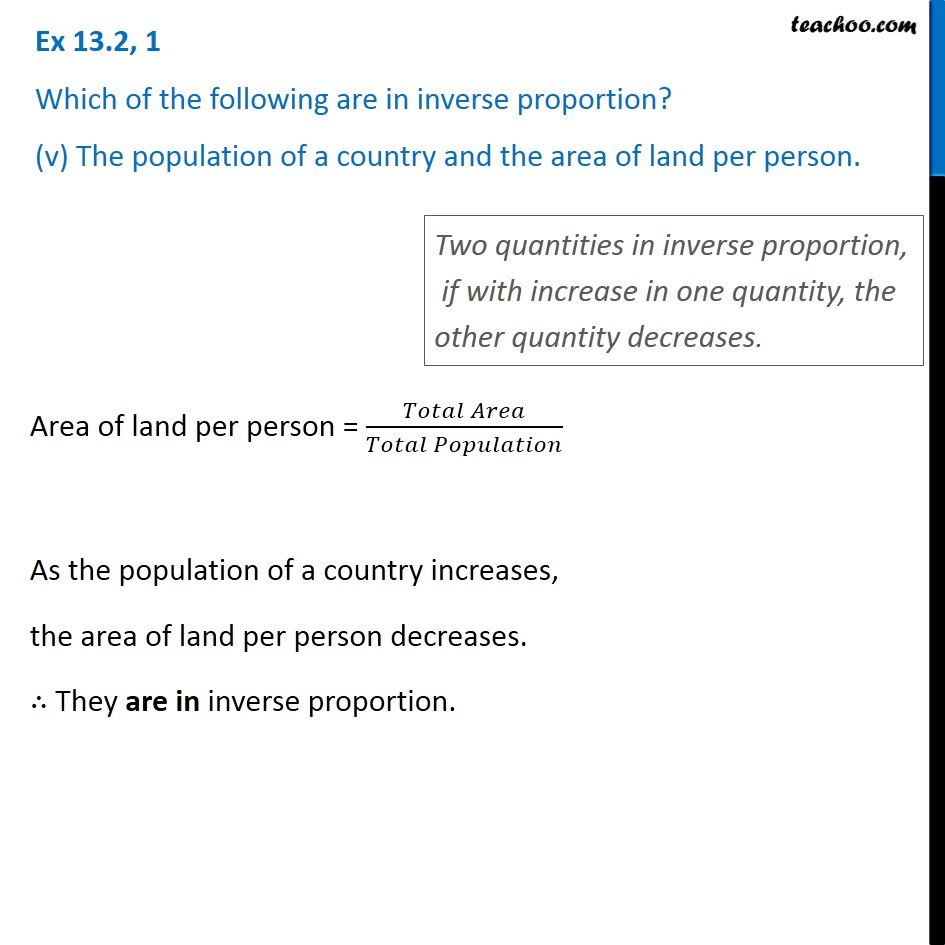Subscribe to our Youtube Channel - https://you.tube/teachoo

1. Chapter 13 Class 8 Direct and Inverse Proportions
2. Serial order wise
3. Ex 13.2

Transcript

Ex 13.2, 1 Which of the following are in inverse proportion? (i) The number of workers on a job and the time to complete the job. Two quantities in inverse proportion, if with increase in one quantity, the other quantity decreases. As the number of workers on a job increase, Time taken to complete the job decreases. ∴ They are in inverse proportion. 1 worker complete work in 5 days 5 workers complete work in 1 day Ex 13.2, 1 Which of the following are in inverse proportion? (ii) The time taken for a journey and the distance travelled in a uniform speed. Two quantities in inverse proportion, if with increase in one quantity, the other quantity decreases. As the time taken for a journey increases, Distances travelled also increases. ∴ Time and distance are not in inverse proportion. As the time taken for a journey increases, Distances travelled also increases. ∴ Time and distance are not in inverse proportion. Two quantities in inverse proportion, if with increase in one quantity, the other quantity decreases. As area of cultivated land increases, The crop harvested also increases. ∴ They are not in inverse proportion. Ex 13.2, 1 Which of the following are in inverse proportion? (iv) The time taken for a fixed journey and the speed of the vehicle. Two quantities in inverse proportion, if with increase in one quantity, the other quantity decreases. As speed of the vehicle increases, The time taken for a journey decreases ∴ Speed and time are in inverse proportion. Ex 13.2, 1 Which of the following are in inverse proportion? (iv) The time taken for a fixed journey and the speed of the vehicle. Two quantities in inverse proportion, if with increase in one quantity, the other quantity decreases. As speed of the vehicle increases, The time taken for a journey decreases ∴ Speed and time are in inverse proportion. As speed of the vehicle increases, The time taken for a journey decreases ∴ Speed and time are in inverse proportion. Two quantities in inverse proportion, if with increase in one quantity, the other quantity decreases. Area of land per person = (𝑇𝑜𝑡𝑎𝑙 𝐴𝑟𝑒𝑎)/(𝑇𝑜𝑡𝑎𝑙 𝑃𝑜𝑝𝑢𝑙𝑎𝑡𝑖𝑜𝑛) As the population of a country increases, the area of land per person decreases. ∴ They are in inverse proportion.

Ex 13.2